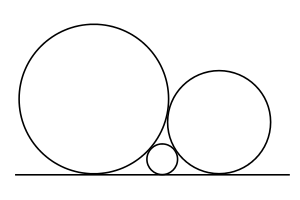#### You may also like### Kissing

Two perpendicular lines are tangential to two identical circles that touch. What is the largest circle that can be placed in between the two lines and the two circles and how would you construct it?### Logosquares

Ten squares form regular rings either with adjacent or opposite vertices touching. Calculate the inner and outer radii of the rings that surround the squares.### Just Touching

Three semi-circles have a common diameter, each touches the other two and two lie inside the biggest one. What is the radius of the circle that touches all three semi-circles?

# Baby Circle

##### Age 16 to 18Challenge LevelA circle with radius 1 and a circle with radius 2 touch at a point. A third circle fits between these two circles so that all three touch each other and all three have a common tangent. What is the radius of the smallest circle?